cancel
Showing results for
Did you mean: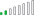Resolver II

## Average by family

Hi,

i've a dataset with an id and a family.

i want to calculate the kms average by id so far so good

My issue is when i want to display in front of each id, the family average like below.i don't find the dax code to stay at the family level

1 ACCEPTED SOLUTIONResolver II

Thanks @Arul

I finally managed to display what i want

it was the wrong columns i assume...

i now display the column of the calculate table, with the column of the vehicle just be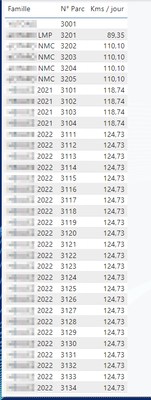For those who are inserested, if you want to display the value in a card you muste create a measure :

``````kms quotidien moyen famille =
LOOKUPVALUE('Données par Famille'[Kms / jour], 'Données par Famille'[Famille], SELECTEDVALUE('Paramètres Véhicules'[Famille]))``````

10 REPLIES 10Resolver II

Hello @Arul

they are indeed in different tables, they are related by the id.Resolver II

Here is the data modelin 1 you can see the links between the id and the family.

in 2 i tried to sumarize the date by family in a separated table. i also tried a lookupvalue, but without success.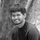Super User

What are all the columns that you are using in your visual?

Thanks,

Arul

If I answered your question then mark my post as a solution and a kudo would be appreciated.Resolver II

i use

'Paramètres Véhicules'[Famille], 'Paramètres Véhicules'[N° Parc] and the measure

``````kms quotidien moyen famille =
CALCULATE(
AVERAGE('daily_aggregate_final'[max_distance])
,ALLEXCEPT('Paramètres Véhicules', 'Données par Famille'[Famille])
)``````Super User

Am asking the columns and measures used in the visual.

Thanks,

Arul

If I answered your question then mark my post as a solution and a kudo would be appreciated.Resolver II

Thanks @Arul

I finally managed to display what i want

it was the wrong columns i assume...

i now display the column of the calculate table, with the column of the vehicle just beFor those who are inserested, if you want to display the value in a card you muste create a measure :

``````kms quotidien moyen famille =
LOOKUPVALUE('Données par Famille'[Kms / jour], 'Données par Famille'[Famille], SELECTEDVALUE('Paramètres Véhicules'[Famille]))``````Resolver II

i'm not sure that i well understood the question, but here is a screenshot of my visual :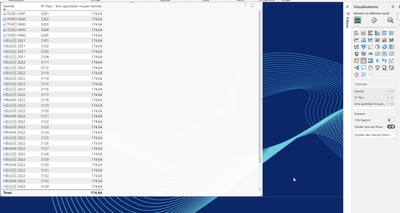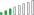Resolver III

Hello, see the formulas below and the outcomes in the image:``````KMS Avg:=AVERAGE([Kms Average])

KMS Family Avg.:=CALCULATE([KMS Avg],FILTER(ALLSELECTED('Table1'[Famille]),'Table1'[Famille]=MAX(Table1[Famille])))

KMS Family Avg v2.:=CALCULATE([KMS Avg],ALLEXCEPT('Table1','Table1'[Famille]))
``````

, but the formula you want is the v2:

``KMS Family Avg v2.:=CALCULATE([KMS Avg],ALLEXCEPT('Table1','Table1'[Famille]))``Resolver II

Hello @BrianConnelly ,

Thanks for your answer, unfortunately, when i display the measure in a table, the average is recalculed by id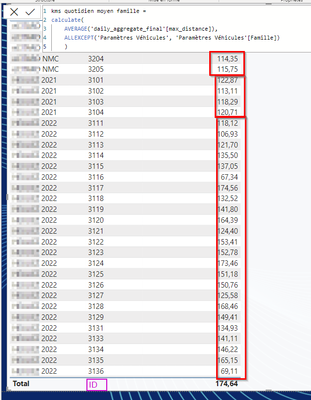Super User

Are the ID column and Famille column comes from different table? If yes, do they have reltionship and what is it?

Thanks,

Arul

If I answered your question then mark my post as a solution and a kudo would be appreciated.